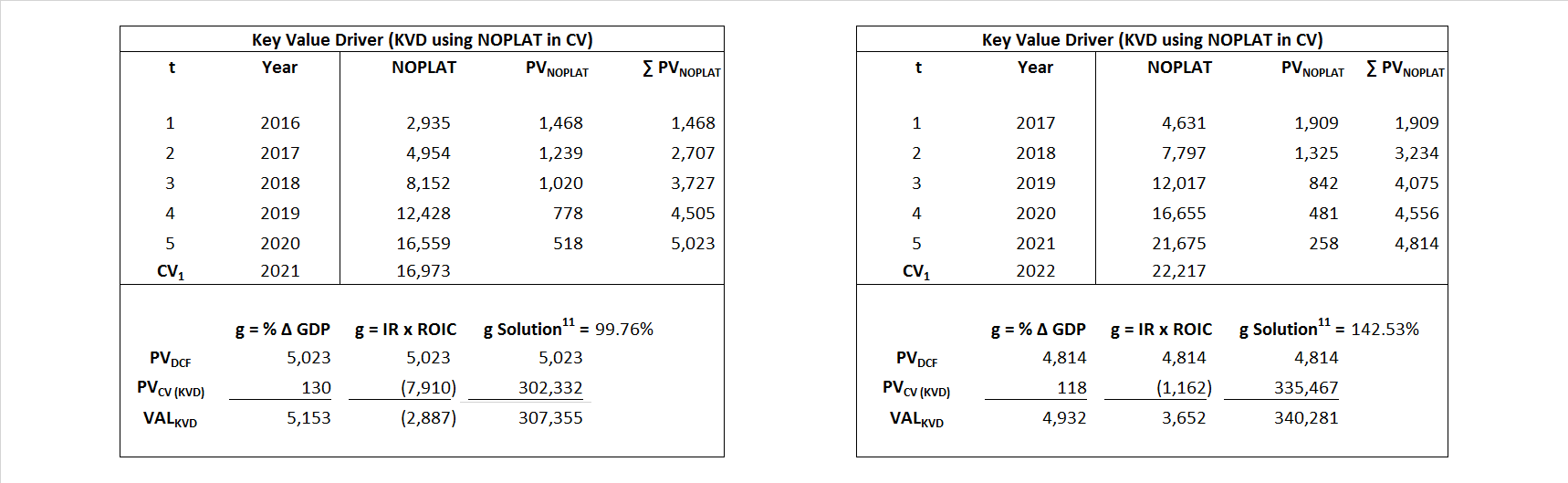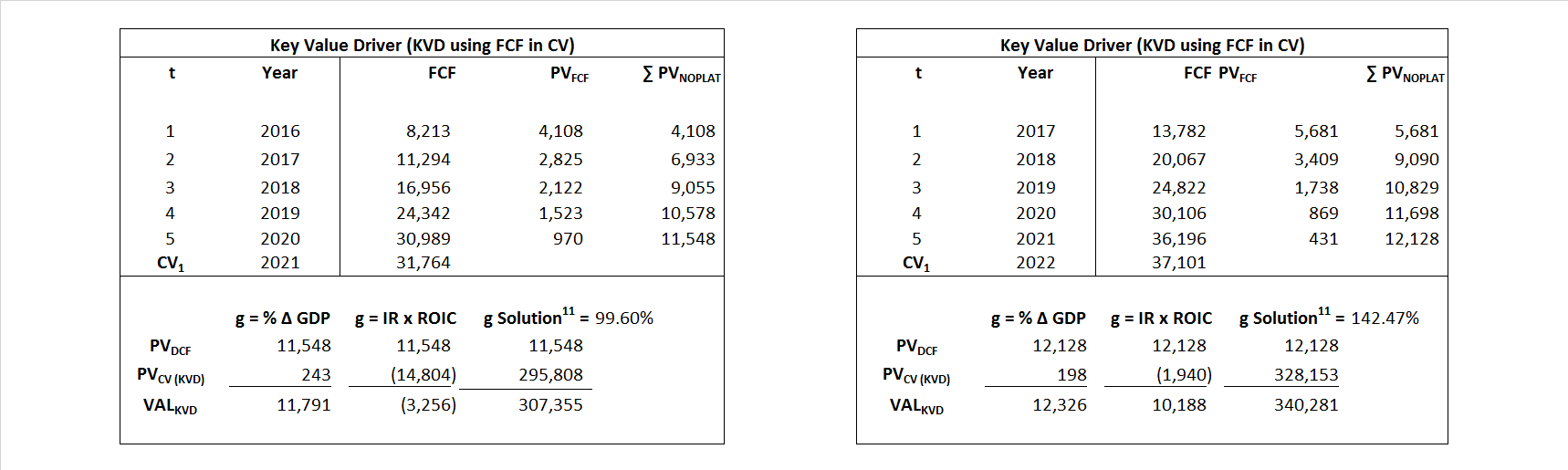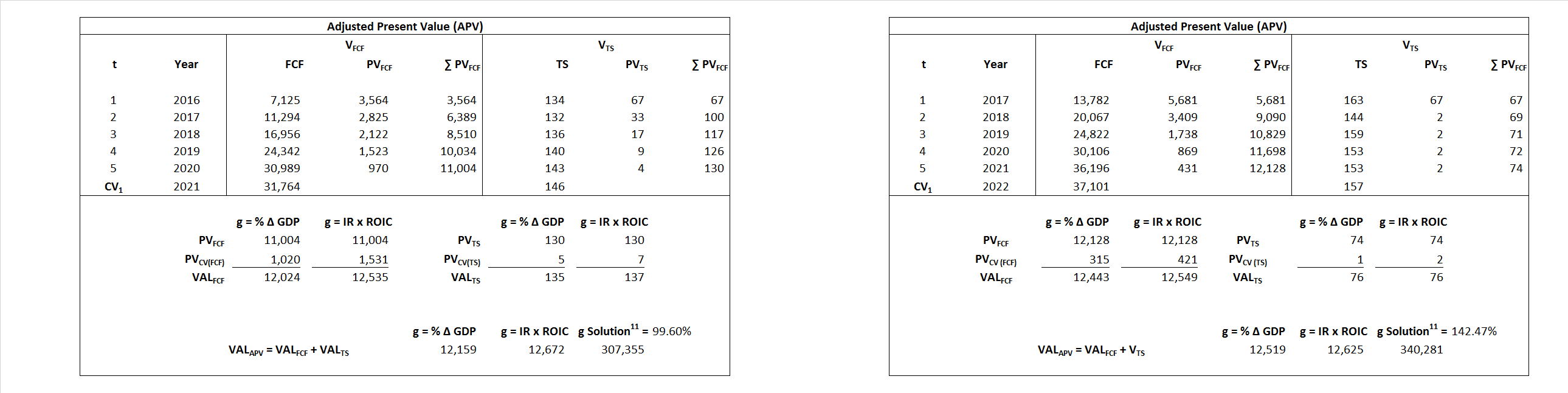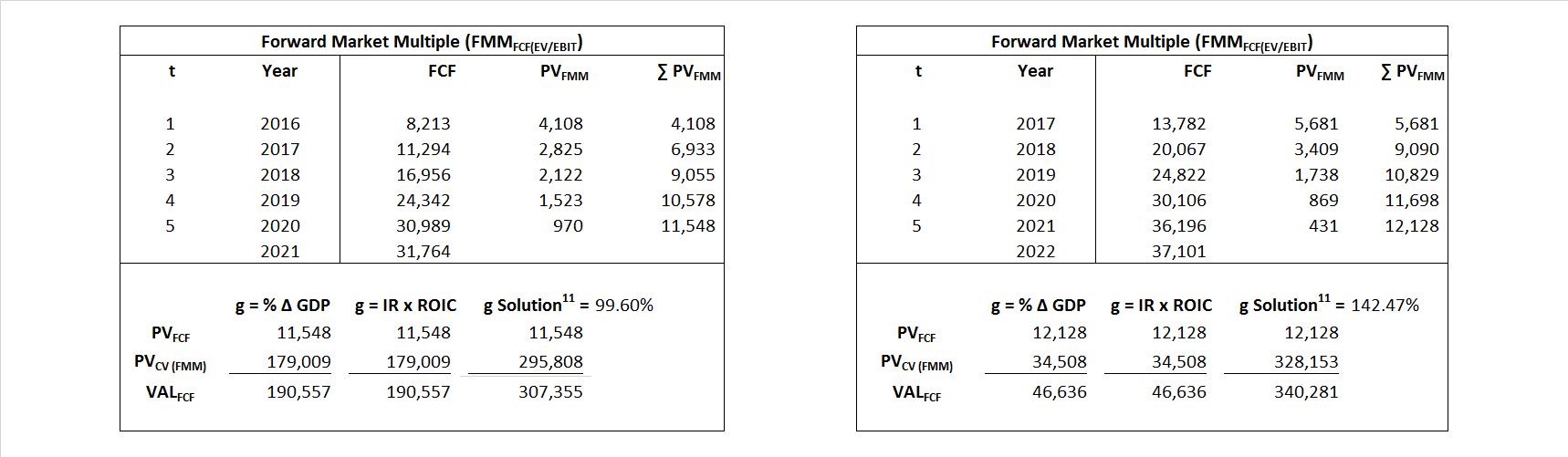# Amazon

## Analyst Listing

The following analysts provide coverage for the subject firm as of May 2016:

 Broker Analyst Analyst Email Raymond James Aaron Kessler aaron.kessler@raymondjames.com Jefferies Brian Pitz bpitz@jefferies.com Bernstein Research Carlos Kirjner carlos.kirjner@bernstein.com Monness Crespi Hardt Cengiz M. Cakmak jcakmak@mchny.com BGC Financial, L.P. Colin W. Gillis cgillis@bgcpartners.com The Benchmark Company, LLC Daniel L. Kurnos dkurnos@benchmarkcompany.com BMO Capital Markets Daniel Salmon dan.salmon@bmo.com KeyBanc Capital Markets Edward Yruma eyruma@key.com Burke and Quick Partners, LLC Frederick W. Moran fmoran@bqpartners.com Piper Jaffray Gene Munster gene.a.munster@pjc.com Atlantic Equities James Cordwell j.cordwell@atlantic-equities.com Oppenheimer Jason Helfstein jason.helfstein@opco.com Cowen & Company John Blackledge john.blackledge@cowen.com Daiwa Securities Co. Ltd. Kazuya Nishimura kazuya.nishimura@us.daiwacm.com Evercore ISI Ken Sena ken.sena@evercoreisi.com Needham Kerry Rice krice@needhamco.com RBC Capital Markets Mark S. Mahaney mark.mahaney@rbccm.com Canaccord Genuity Michael Graham mgraham@canaccordgenuity.com Wedbush Securities Michael Pachter michael.pachter@wedbush.com Mizuho Securities USA Neil A. Doshi neil.doshi@us.mizuho-sc.com Nomura Research Robert S. Drbul robert.drbul@nomura.com SunTrust Robinson Humphrey Robert S. Peck robert.peck@suntrust.com JMP Securities Ronald V. Josey rjosey@jmpsecurities.com Deutsche Bank Research Ross Sandler ross.sandler@db.com Wolfe Research Scott Mushkin smushkin@wolferesearch.com Stifel Nicolaus Scott W. Devitt swdevitt@stifel.com FBN Securities Shebly Seyrafi sseyrafi@fbnsecurities.com Susquehanna Financial Group Shyam Patil shyam.patil@sig.com Credit Suisse Stephen Ju stephen.ju@credit-suisse.com Axiom Capital Victor Anthony vanthony@axiomcapital.com Cantor Fitzgerald Youssef H. Squali ysquali@cantor.com

## Primary Input Data## Derived Input Data

### Equational Form

Net Operating Profit Less Adjusted Taxes NOPLAT   1,110  2,829$NOPLAT\, =\, EBIT\, x\, (1 \,-\, Avg \,\,Tax\,\, Rate\,\, on\,\, EBIT)$
Free Cash Flow FCF  7,331  9,706$FCF\,=NOPLAT\,+\,Non-Cash\,Expenses-\Delta NWC\,-\,NCS$
Tax Shield TS  282  182$TS\,=\,Interest\,\,Paid\,\,x\,\, Avg \,\,Tax\,\,Rate\,\, on\,\, Pre-Tax\,\, Income$
Invested Capital IC  33,806  42,206$IC\,=\,Fixed\,\,Operating\,\,Assets\,\,+\,\,Net\,\, Working\,\, Capital$
Return on Invested Capital ROIC 3.28% 6.70%$ROIC\,=\,\frac { NOPLAT }{ IC }$
Net Investment NetInv  11,836  15,882$NetInv\,=\,{ {IC}_{1}}-{{IC}_{0}}+Depreciation$
Investment Rate IR 1066.74% 561.31%$IR\,=\,\frac {NetInv}{NOPLAT}$
Weighted Average Cost of Capital WACCMarket 99.94% 142.61%$WACC\,=\,\frac { E }{ V } { R }_{ E }\,+\,\frac { P }{ V } { R }_{ P }\,+\,\frac { D }{ V } { R }_{ D }\left( 1- Avg\,\, Tax\,\,Rate\,\,on\,\,Pre-Tax\,\,Income \right)$
WACCBook  8.69% 9.31%
Enterprise value EVMarket  307,355  340,281$EV\,=\,Market\,\,Cap\,\,Equity\,+\,\,Long\,\,Term\,\,Debt\,-\,Cash$
EVBook  306,771  339,401
EV/EBIT Multiple$\frac{EV_{Market}}{EBIT}$  180.06  78.17$EV/EBIT\,=\,\frac { EV}{ EBIT}$
Long-Run Growth g = IR x ROIC
35.01% 37.63% Long-run growth rates of the income variable  are used in the Continuing Value portion of the valuation models.
g = %$\Delta$ GDP 2.50% 2.50%

## Valuation Model Outcomes

The outcomes presented in this study are the result of original input data, derived data, and synthesized inputs and, depending on the equational form of any particular valuation model, may result in irrelevant or implausible results.  For example, in the event WACC < g, the value of this term, often found in the denominator of an equation’s continuation value term, will be expressly negative and may result in a negative overall valuation for the firm.  In the event of a WACC < g relation, the model form as applied to the subject firm offers an irrelevant outcome.

### Equational form

Key Value Driver (NOPLAT) KVD (NOPLAT)${ Value }_{ DCF/KVD }=\sum { \frac { NOPLAT_{ t } }{ { \left( 1+WACC \right) }^{ t } } +\frac { \frac { { NOPLAT }_{ 1 }\left( 1-\frac { g }{ ROIC } \right) }{ WACC-g } }{ { \left( 1+WACC \right) }^{ t } } }$Key Value Driver (FCF) KVD (FCF)${ Value }_{ DCF/KVD }=\sum { \frac { FCF_{ t } }{ { \left( 1+WACC \right) }^{ t } } +\frac { \frac { { NOPLAT }_{ 1 }\left( 1-\frac { g }{ ROIC } \right) }{ WACC-g } }{ { \left( 1+WACC \right) }^{ t } } }$Free Cash Flow FCF${ Value }_{ DCF/FCF }=\sum { \frac { FCF_{ t } }{ { \left( 1+WACC \right) }^{ t } } +\frac { \frac { { FCF }_{ 1 }}{ WACC-g } }{ { \left( 1+WACC \right) }^{ t } } }$Economic Profit ECON π${ Value }_{ { ECON\pi } }= I{ C }_{ 0 }+\sum { \frac { { IC }_{ t-1 }(ROI{ C }_{t}-WAC{C}_{t}) }{ { \left( 1+WACC \right) }^{ t } }+ \frac {\frac { I{C}_{0}\ x\ (ROI{C}_{1}\ -\ WAC{C}_{1}) }{ WACC-g } }{ { \left( 1+WACC \right) }^{ t } } }$Adjusted Present Value APV${ Value }_{ APV }=\sum { \frac { FCF_{ t } }{ { \left( 1+{ k }_{ u } \right) }^{ t } } +\frac { \frac { { FCF }_{ 1 }}{ { k }_{ u }-g } }{ { \left( 1+{ k }_{ u } \right) }^{ t } } } +\sum { \frac { { TS }_{ t } }{ { \left( 1+{ k }_{ tax } \right) }^{ t } } +\frac { \frac { { TS }_{ 1 }}{ { k }_{ tax }-g } }{ { \left( 1+{ k }_{ tax } \right) }^{ t } } }$Forward Market Multiple FMM${ Value }_{ DCF/FMM}=\sum { \frac { FCF_{ t } }{ { \left( 1+WACC \right) }^{ t } } +\frac { { EBIT }_{ 1 }\,{x}\,{FMM}}{ { \left( 1+WACC \right) }^{ t } } }{\,\,\,; \,\,FMM\,=\,\frac{{EV}_{t=0}}{{EBIT}_{t=0}}}$